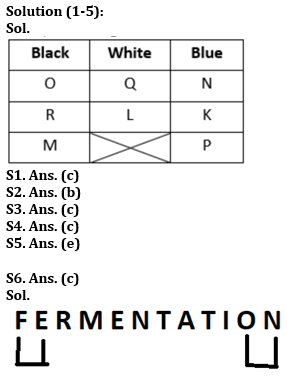Latest Banking jobs   »   Reasoning Ability Quiz For FCI Phase...

# Reasoning Ability Quiz For FCI Phase I 2023- 8th January

Direction (1-5): Read the following alphanumeric series carefully and answer the questions given below.
Eight people K, L, M, N, O, P, Q and R like either of the three colours i.e., Black, White, and Blue. At least two and at most three of them like each colour. O and R like the same colour but not White. N neither likes the same colour as O nor White. Q and L like the same colour but not Blue. M neither likes White nor the same colour as N. K likes the same colour as N. P neither like Black nor White colour.

Q1. Who amongst the following likes Blue colour?
(a) O
(b) R
(c) P
(d) M
(e) None of these

Q2. Who amongst the following likes the same colour as M likes?
(a) P
(b) O
(c) K
(d) N
(e) None of these

Q3. Who amongst the following likes Black colour?
(a) N and K
(b) N, Q and L
(c) O, R and M
(d) O and P
(e) None of these

Q4. Which amongst the following statement is incorrect?
I. O and R like Black colour.
II. Only two people like the Blue colour.
III. K likes the same colour as P likes.
(a) Only III
(b) Only I
(c) Only II
(d) Only I and II
(e) None is correct

Q5. Four of the following five are alike in a certain way and hence form a group. Which is the one that does not belong to that group?
(a) O, M
(b) K, P
(c) Q, L
(d) N, K
(e) P, M

Q6. How many such pairs of letters are there in the word ‘FERMENTATION’ each of which has as many letters between them in the word as in the English alphabet (Both forward and backward)?
(a) None
(b) One
(c) Two
(d) Three
(e) None of these

Q7. How many independent words can ‘MAGNETIC’ be divided into without changing the order of the letters and using each letter only once?
(a) More than three
(b) One
(c) Two
(d) Three
(e) None of these

Q8. In a certain code language, if ‘EMBARK’ is coded as ‘VNYZIP’ and ‘FILMS’ is coded as ‘URONH’, then what is the code for ‘MOBILE’?
(a) NLYRVO
(b) NLYROV
(c) NLYORV
(d) NLYRPV
(e) None of these

Q9. In a certain code language, if ‘PROJECT’ is coded as ‘NPMHCAR’ and ‘OPENING’ is coded as ‘MNCLGLE’, then what is the code for ‘LUXURY’?
(a) JVSSPW
(b) JSVPSW
(c) JSVSWP
(d) JSVSPW
(e) None of these

Q10. If all the letters in the word ‘OFFICER’ are arranged in alphabetical order from left to right in such a way that vowels are arranged first followed by consonants, then how many letters are there in between O and R after the arrangement (repetition of letters should also be considered)?
(a) More than three
(b) One
(c) Two
(d) Three
(e) None of these

Direction (11-15): Study the following data carefully and answer the questions accordingly.

695 523 724 476 367 936

Q11. If 1 is added to the last digit of each of the numbers, then how many numbers thus formed will be divisible by 3?
(a) One
(b) Four
(c) Two
(d) Three
(e) None of these

Q12. If all the digits in each of the numbers are arranged in ascending order within the number, then how many numbers will remain unchanged?
(a) One
(b) Five
(c) Three
(d) Four
(e) None of these

Q13. If all the digits in each of the numbers are arranged in descending order within the number, then what will be the difference between the second-highest and the second-lowest number?
(a) 252
(b) 195
(c) 289
(d) 221
(e) None

Q14. If the positions of the first and the second digits are interchanged, then what will be the sum of the highest and the lowest number?
(a) 1434
(b) 1199
(c) 1543
(d) 1218
(e) None of these

Q15. What will be the result if the second digit of the second-lowest number is multiplied by the second -lowest digit of the largest number?
(a) 56
(b) 42
(c) 48
(d) 64
(e) None of these

SolutionsS7. Ans. (b)
Sol. The words can be either MAGNET or NET but can’t be both because letters are repeated. Hence, only one word can be formed.

S8. Ans. (b)
Sol. Each letter is replaced by the letter of its opposite face value in the alphabetical series. For example, ‘A’ is replaced by ‘Z’, ‘B’ is replaced by ‘Y’ and so on. Hence, MOBILE is coded as NLYROV.

S9. Ans. (d)
Sol. Each letter is moved two places backward in the alphabetical series. Hence, LUXURY is coded as JSVSPW.

S10. Ans. (d)
Sol. Given word: ‘OFFICER’
After rearranging them in alphabetical order from left to right in such a way that vowels are arranged first followed by consonants: ‘EIOCFFR’
Hence, there are three letters in between O and R after the arrangement.

S11. Ans. (c)
Sol. Given Series – 695 523 724 476 367 936
After adding 1 = 696 524 725 477 368 937
Numbers divisible by 3 = 696 477

S12. Ans. (a)
Sol. Given Series = 695 523 724 476 367 936
After rearrangement = 569 235 247 467 367 369

S13. Ans. (d)
Sol. Given Series – 695 523 724 476 367 936
After rearrangement = 965 532 742 764 763 963
Required difference = 963 – 742 = 221

S14. Ans. (d)
Sol. Given Series – 695 523 724 476 367 936
After interchange= 965 253 274 746 637 396
Required sum = 965 + 253 = 1218

S15. Ans. (b)
Sol. Given Series – 695 523 724 476 367 936
Required resultant = 7 x 6 = 42## FAQs

### Q1. Who amongst the following likes Blue colour?

S1. Ans. (c)

#### Congratulations!